# 現代數學 Modern Mathematics

•### 小二 加減應用題 P.2 Addition and Subtraction Problem

Activity

現代數學 Modern Mathematics

•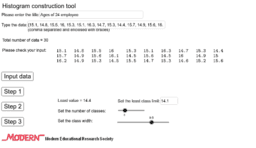### Histogram construction tool

Activity

現代數學 Modern Mathematics

•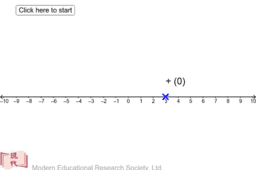Activity

現代數學 Modern Mathematics

•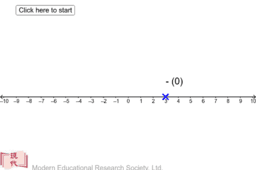### Subtraction of directed numbers

Activity

現代數學 Modern Mathematics

•### Multiplication of directed numbers

Activity

現代數學 Modern Mathematics

•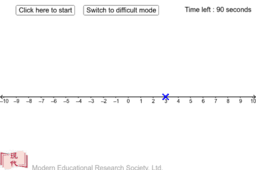### Addition of directed numbers (practice mode)

Activity

現代數學 Modern Mathematics

•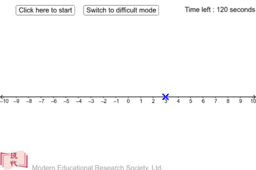### Subtraction of directed numbers (practice mode)

Activity

現代數學 Modern Mathematics

•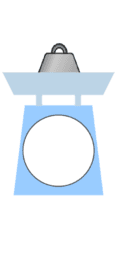### 小三 用磅來稱量物件 P.3 Using a Weighing Scale to Weigh Objects

Activity

現代數學 Modern Mathematics

•### 小三 重量的量度 P.3 Measuring Weights

Activity

現代數學 Modern Mathematics

•### 小五 分數乘法 P.5 Multiplication of Fractions

Activity

現代數學 Modern Mathematics